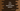# C++ program to print a square hollow pattern# C++ program to print a square hollow pattern :

In this tutorial, we will learn how to print one square hollow pattern in C++ using user input values. This is a square with hollow inside. We will write one program that will take the size of the square and the character to print the square as user inputs. I will show you two different ways to solve it.

## Method 1: Using a for loop :

``````#include <iostream>
using namespace std;

void printHollowSquare(int size, char c)
{
// 5
for (int i = 0; i < size; i++)
{
for (int j = 0; j < size; j++)
{
// 6
if (i == 0 || i == size - 1 || j == 0 || j == size - 1)
{
cout << c << " ";
}
else
{
cout << " "
<< " ";
}
}
cout << '\n';
}
}
int main()
{
// 1
int size;
char c;

// 2
cout << "Enter the size of the square : " << endl; cin >> size;

// 3
cout << "Enter the character to print the square : " << endl; cin >> c;

// 4
printHollowSquare(size, c);

return 0;
}``````

### Explanation :

The commented numbers in the above program denote the step numbers below :

1. Create two variables size and c to store the size of the hollow square and the character to print this square.
2. Ask the user to enter the size of the square. Read it and store it in the size variable.
3. Ask the user to enter the character to print the square. Read and store it in variable c.
4. Call the printHollowSquare method to print the hollow square. This method takes two arguments: The size of the hollow square and the character to print the hollow square.
5. We are using two for loops to print the hollow square. One for loop runs inside another and both will run for size number of times where size is the size of the square.
6. Inside these loops, we are printing the character if it is first row, last row, first column or second column. Else, we are printing one blank space. We are also printing one new line once the inner for loop ends.

### Sample output :

Enter the size of the square : 4 Enter the character to print the square : & & & & & &        & &        & & & & &

Enter the size of the square : 6 Enter the character to print the square : *

*            * *            * *            * *            *

You can print using any character you want.

## Using a while loop :

We can also use one while loop to do the exact same implementation that we did above. It looks like as below :

``````void printHollowSquare(int size, char c)
{
int i = 0;
int j = 0;

while (i < size)
{
j = 0;
while (j < size)
{
if (i == 0 || i == size - 1 || j == 0 || j == size - 1)
{
cout << c << " ";
}
else
{
cout << " "
<< " ";
}
j++;
}
cout << '\n';
i++;
}
}``````

The only changes are that we have initialized two variables i and j. Other than that, everything is the same. It will print the same output as the previous one.

Try to go through the steps and implement it on your system. If you have any queries, drop one comment below.